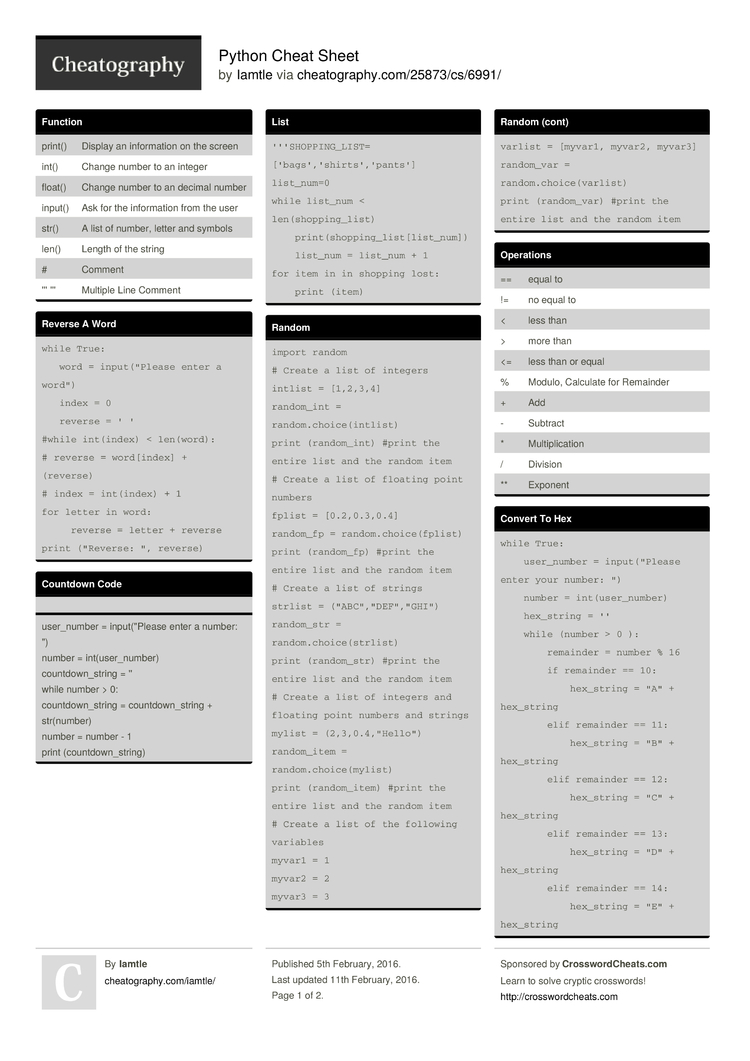# Python Cheat Sheet by Iamtle

### List

 ```'''SHOPPING_LIST=['bags','shirts','pants'] list_num=0 while list_num < len(shopping_list)     print(shopping_list[list_num])     list_num = list_num + 1 for item in in shopping lost:     print (item)```

### Random

 ```import random # Create a list of integers intlist = [1,2,3,4] random_int = random.choice(intlist) print (random_int) #print the entire list and the random item # Create a list of floating point numbers fplist = [0.2,0.3,0.4] random_fp = random.choice(fplist) print (random_fp) #print the entire list and the random item # Create a list of strings strlist = ("ABC","DEF","GHI") random_str = random.choice(strlist) print (random_str) #print the entire list and the random item # Create a list of integers and floating point numbers and strings mylist = (2,3,0.4,"Hello") random_item = random.choice(mylist) print (random_item) #print the entire list and the random item # Create a list of the following variables myvar1 = 1 myvar2 = 2 myvar3 = 3 varlist = [myvar1, myvar2, myvar3] random_var = random.choice(varlist) print (random_var) #print the entire list and the random item```

### Function

 print() Display an inform­ation on the screen int() Change number to an integer float() Change number to an decimal number input() Ask for the inform­ation from the user str() A list of number, letter and symbols len() Length of the string # Comment ''' ''' Multiple Line Comment

### Reverse A Word

 ```while True:    word = input(­"­Please enter a word")    index = 0    reverse = ' ' #while int(index) < len(word): # reverse = word[i­ndex] + (reverse) # index = int(index) + 1 for letter in word:      reverse = letter + reverse print ("Re­verse: ", reverse)```

### Countdown Code

user_n­umber = input(­"­Please enter a number: ")
number = int(us­er_­number)
countd­own­_string = ''
while number > 0:
countd­own­_string = countd­own­_string + str(nu­mber)
number = number - 1
print (count­dow­n_s­tring)

### Area Of The Circle Code

 ```while True:     user_radius = input("What is your radius of a circle? ")     radius = float(user_radius)     pi = float(3.1415)     area = (pi) (radius) * 2     print("The area of the circle", area)```

### Upper and Lower Case

name = "one two"

print (name.u­­p­p­er()) → ONE TWO
print (name.l­­o­w­er()) → one two
print (name.c­­a­p­i­ta­­lize()) → One two
print (name.t­­i­t­le()) → One Two

### Operations

 == equal to != no equal to < less than > more than <= less than or equal % Modulo, Calculate for Remainder + Add - Subtract * Multip­lic­ation / Division ** Exponent

### Convert To Hex

 ```while True:     user_number = input("Please enter your number: ")     number = int(user_number)     hex_string = ''     while (number > 0 ):         remainder = number % 16         if remainder == 10:             hex_string = "A" + hex_string         elif remainder == 11:             hex_string = "B" + hex_string         elif remainder == 12:             hex_string = "C" + hex_string         elif remainder == 13:             hex_string = "D" + hex_string         elif remainder == 14:             hex_string = "E" + hex_string         elif remainder == 15:             hex_string = "F" + hex_string         elif remainder < 10:             hex_string = str(remainder) + hex_string         number = number // 16     print ("Hexadeimal string is 0x"+ hex_string)```

### Multip­lic­ation And String

 ```String * Number Ex. String * 5 = String String String String String String * String = Crash ! Number * Number = Multiply (Math) Ex. 5 * 4 = 20 String ** String = CRASH! Number ** Number = Exponent (Math) Ex. 5**2 = 25 String ** Number = CRASH!```

### Guessing Game

 ```import random game_over = 1 chances = 5 score = 0 word = ['Dog','Cat','Fish','Pig','Elephant'] random_word = random.choice(word) while game_over != 0:     print ("Word:",(word))     guess_word = input("Guess a word: ")          if guess_word == random_word:         print ("That's Correct Guess!")         print ("------------------------------------------------------")         score = score + 100         print ("Score:",(score))         random_word = random.choice(word)     else:         if guess_word != random_word:             chances = chances - 1             print ("Chances Remaining:",chances)             if guess_word in word:                 print ("Sorry,Wrong Choice!")                 print ("------------------------------------------------------")             else:                 print ("Sorry That is not even in the list")                 print ("------------------------------------------------------")         if chances == 0:             game_over = 0             print ("Game Over!")             print ("The word was",(random_word))             print ("Final Score:",(score))```

### Vocabu­larty

 Variable reserved memory locations to store values. This means that when you create a variable you reserve some space in memory String A list of character such as number, letter and symbols Boolean True/False Integer Number Whole Number or Counting Number Syntax Grammar of Python Floating Point Number in Decimal

### Example Of Codes

 2 – integer 2.5 – floating point Print (“Hello”) – string mystring = 123 – variable Print (mystr­­,”­H­i­”,­­2,1.0) -- commas mystr = “Hi” mystr ← variable name “Hi” ← value that can be change print (int(1.5)) → 1 print (int(“2”)) → 2 print (float(1)) → 1.0 Modulo­­/R­e­m­ainder % print (4%2) → 0 print (30%7) → 22 Pages
//media.cheatography.com/storage/thumb/iamtle_python.750.jpg

PDF (recommended)